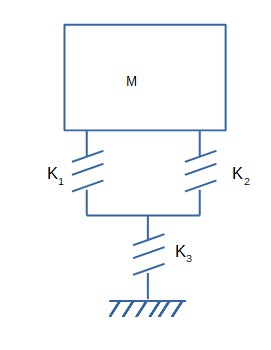# Determine the equivalent stiffness of the system shown in the figure relative to the spatial...

## Question:

Determine the equivalent stiffness of the system shown in the figure relative to the spatial variable y.## Stiffness

Stiffness can be defined as the resistance of the material towards deformation. In springs we can see the stiffness word used more. The thicker the spring is the higher the stiffness and vice versa. Deformation is nothing but the change in the shape from the original shape due to externally applied force.

Become a Study.com member to unlock this answer! Create your account

The stiffness is given by the formula {eq}k=\dfrac{F}{y} {/eq}

F = force

y = deformation

If the force is constant then

{eq}k=\dfrac{1}{y} {/eq}

...Hooke's Law & the Spring Constant: Definition & Equation

from

Chapter 4 / Lesson 19
202K

After watching this video, you will be able to explain what Hooke's Law is and use the equation for Hooke's Law to solve problems. A short quiz will follow.